サイトナビゲーションへ移動

サイドバーへ移動

ここは、本文エリアの先頭です。

# OpenACC

## Optimization of search of neighbor-particle in MPS method by using OpenACC

### 1. MPS method and Search of neighbor-particle

#### (a) Moving Particle Semi-implicit (MPS) method

MPS method is a sort of particle methods used for computation fluid dynamics. It is originally developed for simulating fluid dynamics such as fragmentation of incompressible fluid. Target fluid or objects are divided into particles and each particle interacts with neighbor-particles. Search of neighbor-particles is a main bottleneck of MPS method. We’re researching and developing in-house program.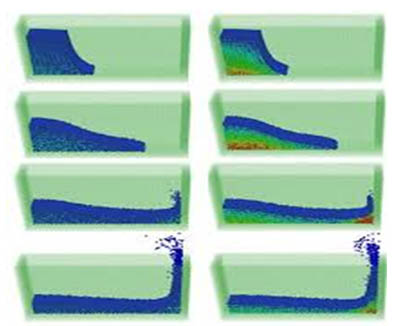Dam-brake simulation

#### (b) Search of neighbor-particle in MPS

MPS performs search of neighbor-part-icle multiple times in each time step. Each particle drifts as time-step progresses and neighbor-particle changes momentarily.

To compute a value of the target partic-le (red particle), it first requires a distance of each neighbor-particle in adjacent buckets. Then, weight of each neighbor-particle is calculated from a distance and weight function. Finally, all the weight is accumulated to center particle. All the particle in simulation area require the above computation.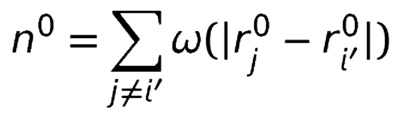Equation of density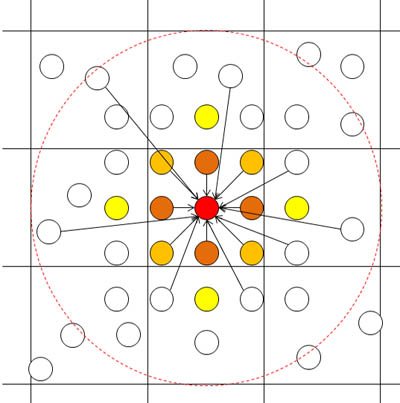Search of neighbor-particle

#### (c) Original Implementation and added OpenACC directives

The following is a pseudo code of search of neighbor-particle. We added OpenACC directives so as to port to GPGPU.### 2. Four optimizations by using OpenACC

OpenACC is an emerging APIs for GPU. It provides compiler directives, runtime library routines, and environment variables. We implement and evaluate the following optimizations.

• Add !\$acc kernels to the do-loop1, !\$acc loop gang to the do-loop2 and !\$acc loop reduction to the do-loop5.
• Sequential addition to global memory.
• Kernel computes weight function of each particle in do-loop5 sequentially. do-loop 2,3,4 run in parallel and each iteration runs in sequential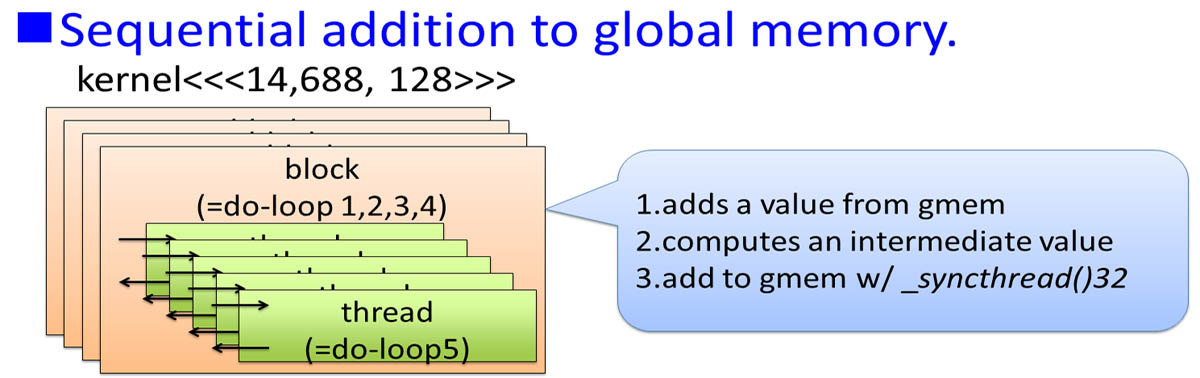#### (b) Add !\$acc parallel loop

• Add !\$acc parallel loop to the do-loop1 instead of !\$acc kernels. We remove all the other directives.
• Reduction with texture cache
• First kernel computes weight function of each particle in do-loop5 sequentially.
• Second kernel uses reduction with an interleaved pairing and loop unroll techniques so as to calculate final value.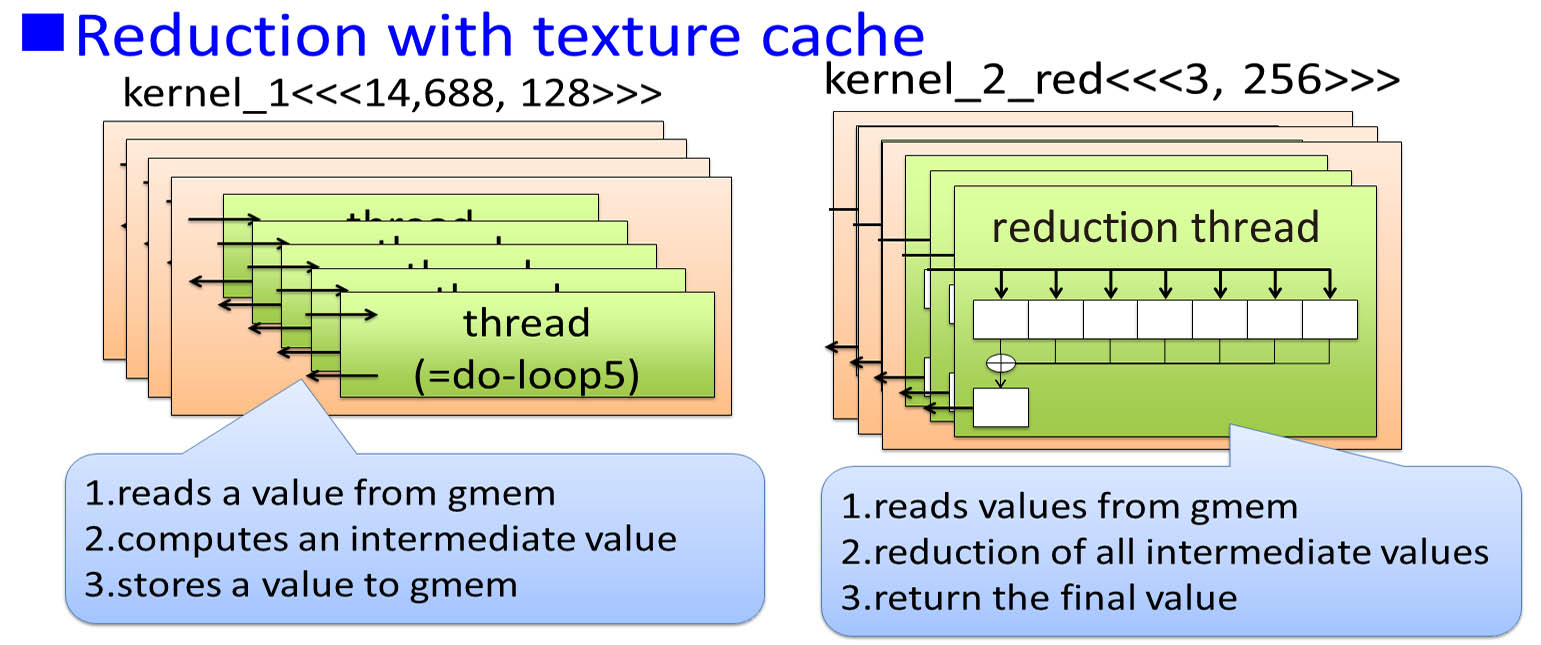#### (c) Merge if-else branch & 4. Remove redundant variables

• Merge a large if-else branch in do-loop5. Directives are the same as “!\$acc parallel loop” optimization.
• Efficient two-level reduction with L2 cache.
• First kernel accumulates intermediate values in parallel. Interleaved pairing and loop unroll are used. Used registers is increased.
• Second kernel is the same as “!\$acc parallel loop”.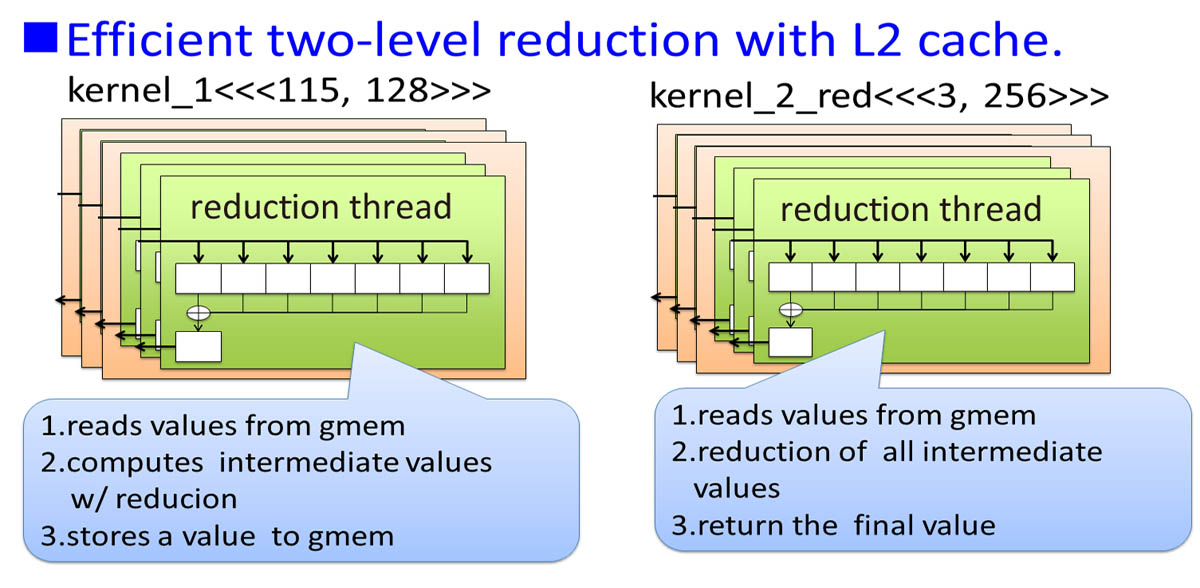### 3. Evaluation on NVIDIA Tesla K20c (Kepler, 706MHz, 2496 CUDA Cores, CC 3.5)

ここは、サイドバー・エリアの先頭です。

# How to use JSS3

 To use JSS3, please refer to "How to use JSS3" page .

# Location

Chofu Aerospace Center
7-44-1 Jindaiji Higashi-machi, Chofu-shi, Tokyo